##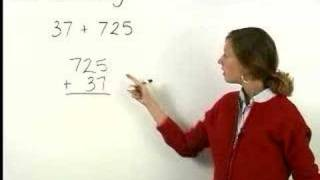By yourteachermathhelp##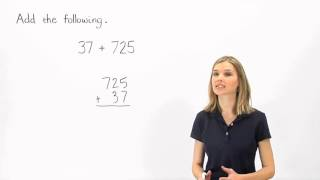By MathHelp.com##By MathHelp.com

Note that Elementary Algebra is also called Introductory Algebra. MathHelp.com offers a comprehensive Elementary Algebra course with a teacher. Perfect for students who are stuck in Elementary Algebra and would rather learn the material on their own and place out instead.# Multiplying fractions by whole numbers 4.NF.B.4 4.NF.4

##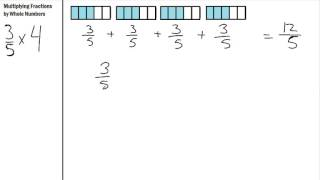By S Hart

Multiplying Fractions by Whole numbers using repeated addition# Adding Fractions with Like Denominators

##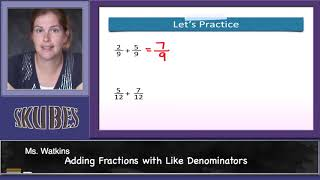By Skubes ed

Understand addition and subtraction of fractions as joining and separating parts referring to the same whole# [4.NF.3a-1.0] Intro to Fraction Addition - Common Core Standard - Word Problem

##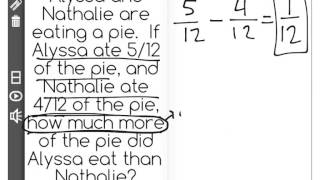By Freckle education

Understand addition and subtraction of fractions as joining and separating parts referring to the same whole# [4.NF.3a-1.0] Intro to Fraction Addition - Common Core Standard

##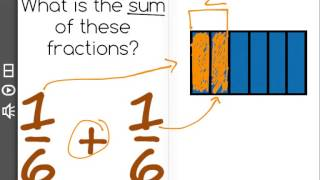By Freckle education

Understand addition and subtraction of fractions as joining and separating parts referring to the same whole# Subtracting Fractions in a Word Problem

##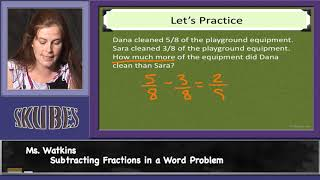By Skubes ed

Solve word problems involving addition and subtraction of fractions referring to the same whole and having like denominators# Multistep Fraction Problems

##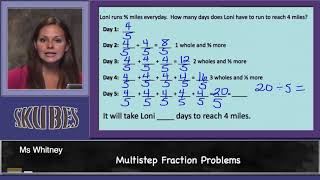By Skubes ed

Solve word problems involving addition and subtraction of fractions referring to the same whole and having like denominators# MULTIPLYING FRACTIONS WORD PROBLEMS

##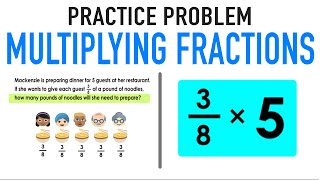By Mashup Math

Solve word problems involving addition and subtraction of fractions referring to the same whole and having like denominators# [4.NF.3a-1.0] Intro to Fraction Addition - Common Core Standard - Word Problem

##By Freckle education

Understand a fraction a/b with a greater than 1 as a sum of fractions 1/b. Understand addition and subtraction of fractions as joining and separating parts referring to the same whole.# [4.NF.3a-1.0] Intro to Fraction Addition - Common Core Standard

##By Freckle education

Understand a fraction a/b with a greater than 1 as a sum of fractions 1/b. Understand addition and subtraction of fractions as joining and separating parts referring to the same whole.# Using Addition to Find Unknown Angles

##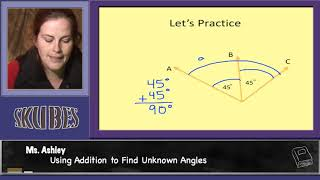By Skubes ed

Recognize angle measure as additive. When an angle is decomposed into non-overlapping parts, the angle measure of the whole is the sum of the angle measures of the parts.# Adding and Subtracting Angles: 4.MD.7

##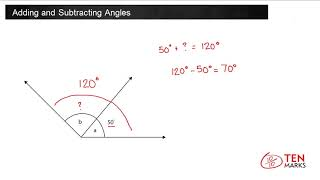By TenmarksAmazon

Recognize angle measure as additive. When an angle is decomposed into non-overlapping parts, the angle measure of the whole is the sum of the angle measures of the parts. Solve addition and subtraction problems to find unknown angles on a diagram in real world and mathematical problems# [4.NF.3d-1.0] Word Problems: Addition - Common Core Standard

##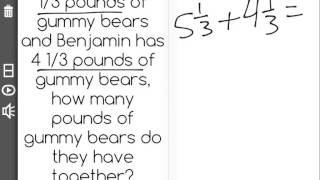By Freckle education

Solve word problems involving addition and subtraction of fractions referring to the same whole and having like denominators, e.g., by using visual fractions models and equations to represent to problem.# Subtracting Fractions from a Whole Number

##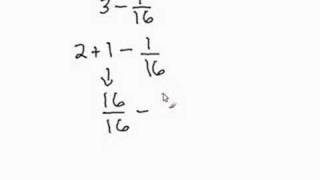By Davitily

In this short video students can learn how to subtract fractions from a whole number. The instructor uses computer software to demonstrate. Only one example is used in this video: 3-1/16.# Multiplying Fractions Part 1a

##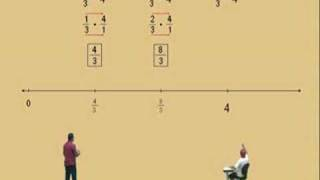By MuchoMath

In this video they show how to multiply fractions in this part they are also using a number line so there is a visual representation. They start with a fraction and multiply by a whole number they show the repeated addition then show it on the number line then they show it as a multiplication problem. They do many more examples. Video is good quality and good for all students as review or initial learning of the concept.# [4.NF.3a-1.0] Intro to Fraction Addition - Common Core Standard - Word Problem

##By Freckle by Renaissance

Understand a fraction a/b with a greater than 1 as a sum of fractions 1/b. Understand addition and subtraction of fractions as joining and separating parts referring to the same whole. Front Row is a free, adaptive, Common Core aligned math program for teachers and students in kindergarten through eighth grade. Front Row allows students to practice math at their own pace - learning advanced concepts when they're ready and receiving remediation when they struggle. Front Row provides teachers with access to a detailed data dashboard and weekly email reports that show which standards are causing students difficulty, what small groups can be formed for interventions, and how their students are progressing in math.# [4.NF.3a-1.0] Intro to Fraction Addition - Common Core Standard

##By Freckle by Renaissance

Understand a fraction a/b with a greater than 1 as a sum of fractions 1/b. Understand addition and subtraction of fractions as joining and separating parts referring to the same whole. Front Row is a free, adaptive, Common Core aligned math program for teachers and students in kindergarten through eighth grade. Front Row allows students to practice math at their own pace - learning advanced concepts when they're ready and receiving remediation when they struggle. Front Row provides teachers with access to a detailed data dashboard and weekly email reports that show which standards are causing students difficulty, what small groups can be formed for interventions, and how their students are progressing in math.# [4.NF.3d-1.0] Word Problems: Addition - Common Core Standard

##By Freckle by Renaissance

Solve word problems involving addition and subtraction of fractions referring to the same whole and having like denominators, e.g., by using visual fractions models and equations to represent to problem. Front Row is a free, adaptive, Common Core aligned math program for teachers and students in kindergarten through eighth grade. Front Row allows students to practice math at their own pace - learning advanced concepts when they're ready and receiving remediation when they struggle. Front Row provides teachers with access to a detailed data dashboard and weekly email reports that show which standards are causing students difficulty, what small groups can be formed for interventions, and how their students are progressing in math.